﻿ Mathematics stock image. Image of maths, science, school - 1341655

# Mathematics.

## Mathematics graph and calculations in black and white

Designers also selected these stock photos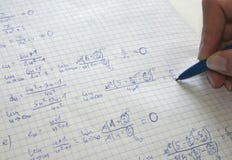Mathematics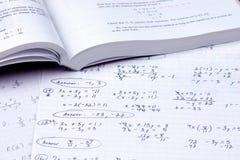Do the math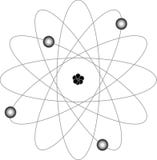Atom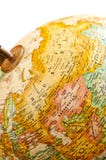Globe - Russia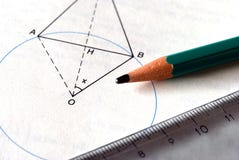Mathematics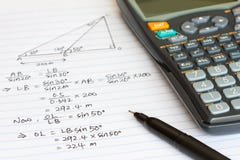Mathematics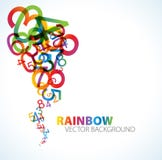Abstract background with numbersReading books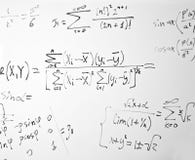Impregnable mathematics
More similar stock images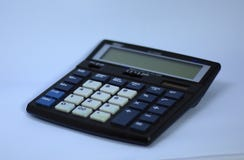A electronic digital calculatorThe Winton Mathematics Gallery at the Science Museum. Designed by Zaha Hadid Architects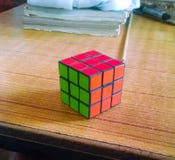Close up of rubik `s cube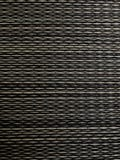Reeds matLearn the art of stacking stones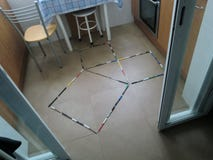Graphical demonstration of the Pythagorean TheoremMathematics numbers in multicolor on a color full backgroundSTEM education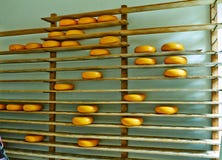Dutch cheese on the shelves in Arnhem Park July
More stock photos from Kmitu's portfolio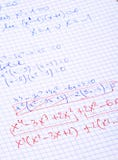Hand written maths calculations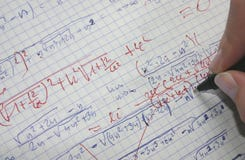Correcting mathsCalculating the profit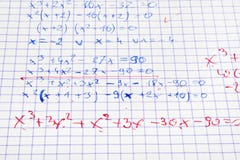Hand written maths calculations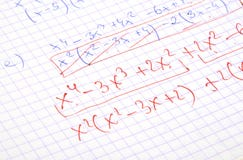Hand written maths calculations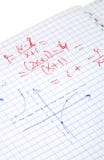Hand written maths calculations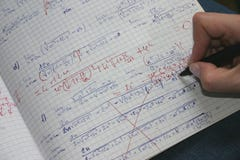Correcting maths #2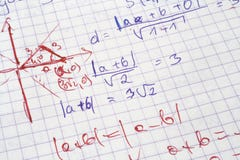MathsCalculating the profit
Related categories